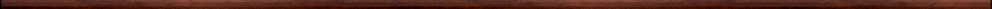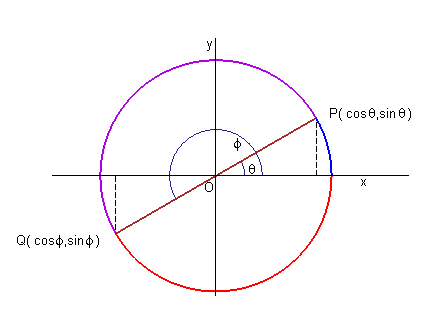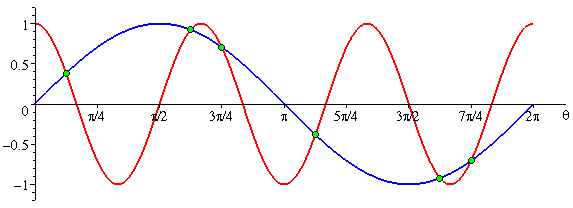Maple worksheets on trigonometric functionsPrecalculus topics:

They are all compatible with Classic Worksheet Maple 10.Trigonometric functions of general angles and real numbers - TRGintro.mws

• Trigonometric functions of angles less than 90 degrees, special values.
• The graph the sine of an acute angle given in degrees.
• Trigonometric functions of general angles and real numbers.
• The graph the sine of an acute angle given in radians.
• A procedure for the animated construction of sine and cosine curves: triganimation.
• The wrapping function.
• The tangent function.
• Calculating values of the sine, cosine and tangent functions in terms of special values.

• Proving trigonometric identities.
• Addition formulas for the sine and cosine functions.
• Examples to illustrate the addition formulas involving the sine and cosine functions.
• Addition formulas for the tangent function.Symmetry formulas.

Double-angle and half-angle formulas - TRGdouble.mws

• Double-angle formulas.
• Half-angle formulas.
• Some trigonometric equations.

Triple angle formulas, converting products to sums and sums to products - TRGprod.mws

• Triple angle formulas.
• Converting sums and differences of sines and cosines to products.
• Some trigonometric equations.

The graphs of sine waves .. amplitude, period and phase shift - TRGgraph.mws

• The amplitude of a sine wave.
• The period of a sine wave.
• Vertical shifts of sine waves.
• Horizontal shifts of sine waves, phase shift.

The reciprocal trigonometric functions - TRGrecip.mws

• The secant, cosecant and cotangent functions.
• Special values and basic identities.

Inverse trigonometric functions and the general solution of trigonometric equations - TRGinv.mws

• The inverse sine function.
• The inverse cosine function.
• The inverse tangent function.
• The general solution of some trigonometric equations using inverse trig functions.Top of page

Main index